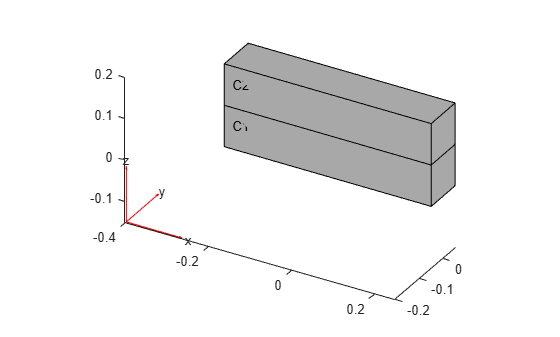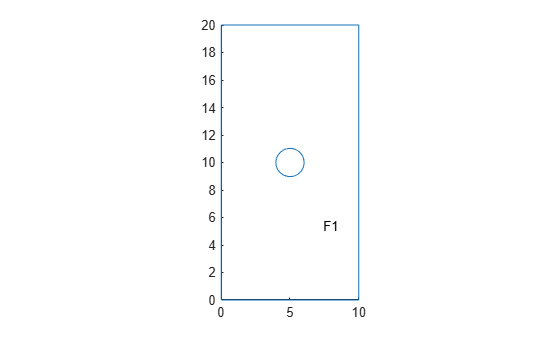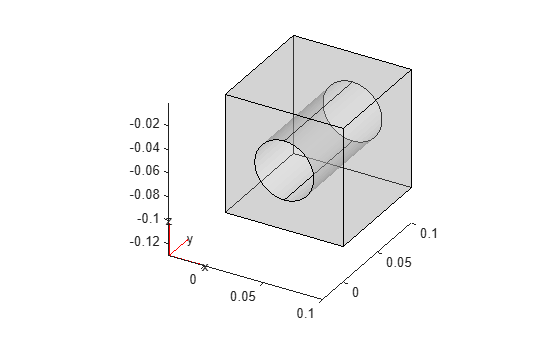# materialProperties

Material properties for structural, thermal, and electromagnetic analysis

Since R2023a

## Description

A `materialProperties` object contains the description of material properties for structural, thermal, and electromagnetic analysis. An `femodel` object contains an array of `materialProperties` objects in its `MaterialProperties` property.

## Creation

### Syntax

``model.MaterialProperties = materialProperties(Name=Value)``
``model.MaterialProperties(RegionID) = materialProperties(Name=Value)``

### Description

example

````model.MaterialProperties = materialProperties(Name=Value)` creates a material properties object and sets properties using one or more name-value arguments. This syntax assigns the specified structural, thermal, or electromagnetic material properties to the entire geometry of the `femodel` object `model`.```

example

````model.MaterialProperties(RegionID) = materialProperties(Name=Value)` assigns material properties to the specified geometry region.```

### Input Arguments

expand all

Cell or face IDs, specified as a vector of positive integers. Find the region IDs using `pdegplot` with the `CellLabels` (3-D) or `FaceLabels` (2-D) value set to `"on"`.

Data Types: `double`

## Properties

expand all

Young's modulus of the material, specified as a positive number, three-element vector of positive numbers (for both 2-D and 3-D geometries), or function handle. Use a function handle to specify Young's modulus that depends on space. For details, see Nonconstant Parameters of Finite Element Model.

Specifying Young's modulus as a three-element vector of positive numbers (or a function handle returning a three-row matrix of positive numbers) means that the material is orthotropic. A matrix of positive numbers specifies the orthotropic Young's modulus along the Cartesian coordinates x, y, and z as [Ex Ey Ez]. For orthotropic materials, you must also specify a shear modulus and Poisson's ratio as three-row matrices of positive numbers or function handles returning three-row matrices of positive numbers.

Data Types: `double`

Poisson's ratio of the material, specified as a positive number, three-element vector of positive numbers (for both 2-D and 3-D geometries), or function handle. Use a function handle to specify Poisson's ratio that depends on space. For details, see Nonconstant Parameters of Finite Element Model. For isotropic materials, the value must be in the range `(0,0.5)`.

Specifying Poisson's ratio as a three-element vector of positive numbers (or a function handle returning a three-row matrix of positive numbers) means that the material is orthotropic. A matrix of positive numbers specifies the orthotropic Poisson's ratio along the Cartesian coordinates x, y, and z as [νxy νyz νxz]. For orthotropic materials, you must also specify Young's modulus and a shear modulus as three-element vectors of positive numbers or function handles returning three-row matrices of positive numbers.

Data Types: `double`

Shear modulus of the material, specified as a three-element vector of positive numbers (for both 2-D and 3-D geometries), or function handle. Specify shear modulus for orthotropic materials. Use a function handle to specify shear modulus that depends on space. For details, see Nonconstant Parameters of Finite Element Model.

Specifying a shear modulus indicates that the material is orthotropic. A matrix of positive numbers specifies the orthotropic shear modulus along the Cartesian coordinates x, y, and z as [Gxy Gyz Gxz]. For orthotropic materials, you must also specify Young's modulus and Poisson's ratio as three-element vectors of positive numbers or function handles returning three-row matrices of positive numbers.

Data Types: `double`

Mass density of the material, specified as a positive number or function handle. Use a function handle to specify mass density that depends on space. For details, see Nonconstant Parameters of Finite Element Model. This property is required when modeling gravitational effects.

Data Types: `double`

Coefficient of thermal expansion of the material, specified as a real number. This property is required for thermal stress analysis. Thermal stress analysis requires the structural model to be static.

Data Types: `double`

Thermal conductivity of the material, specified as a nonnegative number, matrix, or function handle. You can specify thermal conductivity for a steady-state or transient analysis. For orthotropic thermal conductivity, use a thermal conductivity matrix. Use a function handle to specify a thermal conductivity that depends on space, time, or temperature. For details, see Nonconstant Parameters of Finite Element Model.

Data Types: `double` | `function_handle`

Specific heat of the material, specified as a positive number or function handle. Specify this property for a transient thermal conduction analysis. Use a function handle to specify a specific heat that depends on space, time, or temperature. For details, see Nonconstant Parameters of Finite Element Model.

Data Types: `double` | `function_handle`

Hysteretic damping of the material, specified as a nonnegative number. This type of damping is also called structural damping.

Data Types: `double`

Relative permittivity of the material, specified as a number or function handle.

• Use a positive number to specify a relative permittivity for an electrostatic analysis.

• Use a real or complex number to specify a relative permittivity for a harmonic electromagnetic analysis.

• Use a function handle to specify a relative permittivity that depends on the coordinates and, for a harmonic analysis, on the frequency. For details, see Nonconstant Parameters of Finite Element Model.

Data Types: `double` | `function_handle`
Complex Number Support: Yes

Relative permeability of the material, specified as a number or function handle. For a magnetostatic analysis:

• Use a positive number to specify a constant relative permeability.

• Use a function handle to specify a relative permeability that depends on the coordinates, magnetic potential and its gradients, and the norm of the magnetic flux density. For details, see Nonconstant Parameters of Finite Element Model.

For a harmonic analysis:

• Use a real or complex number to specify a constant relative permeability.

• Use a function handle to specify a relative permeability that depends on the coordinates and on the frequency. For details, see Nonconstant Parameters of Finite Element Model.

Data Types: `double` | `function_handle`
Complex Number Support: Yes

Conductivity of the material, specified as a nonnegative number or function handle. Use a function handle to specify a conductivity that depends on the coordinates and, for a harmonic analysis, on the frequency. For details, see Nonconstant Parameters of Finite Element Model.

Data Types: `double` | `function_handle`

## Examples

collapse all

Specify relative permeability for an `femodel` object representing a magnetostatic problem.

Create an `femodel` object for solving a magnetostatic problem, and assign a geometry representing a plate with a hole to the model.

```model = femodel(AnalysisType="magnetostatic", ... Geometry="PlateHolePlanar.stl");```

Plot the geometry with the face labels.

`pdegplot(model.Geometry,FaceLabels="on");`Specify the vacuum permeability value in the SI system of units.

```model.MaterialProperties = materialProperties(RelativePermeability=5000); model.MaterialProperties```
```ans = 1x1 materialProperties array Properties for analysis type: magnetostatic Index RelativePermeability 1 5000 Show all properties ```

Specify Young's modulus, Poisson's ratio, and the mass density for an `femodel` object representing a static structural problem.

Create and plot a bimetallic beam geometry.

```H = 0.1; gm = multicuboid(0.5,0.1,[H,H],Zoffset=[0,H]); pdegplot(gm,CellLabels="on");```Create an `femodel` object for solving a static structural problem, and assign the geometry to the model.

```model = femodel(AnalysisType="structuralStatic", ... Geometry=gm);```

Specify different values of Young's modulus, Poisson's ratio, and the mass density for cells 1 and 2.

```model.MaterialProperties(1) = materialProperties(YoungsModulus=210e9, ... PoissonsRatio=0.28, ... MassDensity=1.3e-5); model.MaterialProperties(2) = materialProperties(YoungsModulus=110e9, ... PoissonsRatio=0.37, ... MassDensity=2.4e-5); model.MaterialProperties```
```ans = 1x2 materialProperties array Properties for analysis type: structuralStatic Index CTE PoissonsRatio YoungsModulus MassDensity ShearModulus 1 [] 0.2800 2.1000e+11 1.3000e-05 [] 2 [] 0.3700 1.1000e+11 2.4000e-05 [] Show all properties ```

Use function handles to specify a thermal conductivity that depends on temperature and a specific heat that depends on coordinates.

Create a rectangular geometry.

`gm = decsg([3 4 -1.5 1.5 1.5 -1.5 0 0 .2 .2]');`

Create an `femodel` object for solving a transient thermal problem, and assign the geometry to the model.

```model = femodel(AnalysisType="thermalTransient", ... Geometry=gm);```

Specify the thermal conductivity as a linear function of temperature, k = 40 + 0.003T.

`k = @(location,state)40 + 0.003*state.u;`

Specify the specific heat as a linear function of the y-coordinate, cp = 500y.

`cp = @(location,state)500*location.y;`

Specify the thermal conductivity, mass density, and specific heat of the material.

```model.MaterialProperties = materialProperties(ThermalConductivity=k, ... MassDensity=2.7*10^(-6), ... SpecificHeat=cp); model.MaterialProperties```
```ans = 1x1 materialProperties array Properties for analysis type: thermalTransient Index ThermalConductivity MassDensity SpecificHeat 1 @(location,state)40+0.003*state.u 2.7000e-06 @(location,state)500*location.y Show all properties ```

Use a function handle to specify a relative permeability that depends on the magnetic flux density.

Create an `femodel` object for solving a magnetostatic problem, and assign a geometry representing a plate with a hole to the model.

```model = femodel(AnalysisType="magnetostatic", ... Geometry="PlateHolePlanar.stl");```

Plot the geometry with the face labels.

`pdegplot(model.Geometry,FaceLabels="on");`Specify the vacuum permeability value in the SI system of units.

`model.VacuumPermeability = 1.2566370614e-6;`

Specify the data for the magnetic flux density, `B`, and the corresponding magnetic field strength, `H`.

```B = [0 .3 .8 1.12 1.32 1.46 1.54 1.61875 1.74]; H = [0 29.8 79.6 159.2 318.31 795.8 1591.6 3376.7 7957.8];```

From the data for `B` and `H`, interpolate the `H(B)` dependency using the modified Akima cubic Hermite interpolation method.

```HofB = griddedInterpolant(B,H,"makima","linear"); muR = @(B) B./HofB(B)/mu0;```

Specify the relative permeability that depends on the magnetic flux density.

```model.MaterialProperties = materialProperties(RelativePermeability=muR); model.MaterialProperties```
```ans = 1x1 materialProperties array Properties for analysis type: magnetostatic Index RelativePermeability 1 @(B)B./HofB(B)/mu0 Show all properties ```

Use vector values to specify Young's modulus, Poisson's ratio, and shear modulus representing an orthotropic material.

Create an `femodel` object for solving a static structural problem, and assign a geometry representing a cube with a cylindrical hole to the model.

```model = femodel(AnalysisType="structuralStatic", ... Geometry="BlockWithHole.step");```

Plot the geometry.

`pdegplot(model.Geometry,FaceAlpha=0.3);`Specify Young's modulus, Poisson's ratio, shear modulus, and the mass density. For an orthotropic material, all parameters, except the mass density, must be 1-by-3 vector of positive numbers.

```E = [22E9,9.8E9,22E9]; nu = [0.28,0.28,0.11]; G = [3.5E9,3.5E9,2.5E9]; rho = 1.3e-5; model.MaterialProperties = materialProperties(YoungsModulus=E, ... PoissonsRatio=nu, ... ShearModulus=G, ... MassDensity=rho); model.MaterialProperties```
```ans = 1x1 materialProperties array Properties for analysis type: structuralStatic Index CTE PoissonsRatio YoungsModulus MassDensity ShearModulus 1 [] [0.2800 0.2800 0.1100] [2.2000e+10 9.8000e+09 2.2000e+10] 1.3000e-05 [3.5000e+09 3.5000e+09 2.5000e+09] Show all properties ```

## Version History

Introduced in R2023a